Goseeko blog Learning beyond college, Students platform for life skills. 2022-05-25T12:06:44Z https://www.goseeko.com/blog/feed/atom/ WordPress https://www.goseeko.com/blog/wp-content/uploads/2021/09/GoSeeko_Stacked-logo-01.png Bhumika <![CDATA[What are the Advantages and disadvantages of OOPs?￼]]> https://www.goseeko.com/blog/?p=7970 2022-05-25T12:06:44Z 2022-05-25T12:06:38Z Object-Oriented Programming is a programming language that is used to create programmes.

The post What are the Advantages and disadvantages of OOPs?￼ appeared first on Goseeko blog.

]]>
Object-Oriented Programming (OOPs) is a programming language that is use to create programmes. It breaks the programme based on the objects it includes, as the name says. In addition, the ideas of class, object, polymorphism, abstraction, encapsulation, and inheritance are all addressed. Its goal is to connect data and functions in order to combine them.

Objective C, Perl, Java, Python, Modula, Ada, Simula, C++, Smalltalk, and certain Common Lisp Object Standard are examples of well-known object-oriented language.

## Advantages of OOPs

• Instead of needing to start writing code from scratch, we may build programmes from standard functioning modules that communicate with one another, saving time and increasing productivity. The OOP language allows us to divide the programme down into small problems that can solved quickly (one object at a time).
• Secondly, The new technology promises increased developer productivity, higher software quality, and lower maintenance costs.
• From tiny to large systems, OOP systems can be simply upgraded.
• Multiple instances of objects can coexist without interfering with one other, and it is very simple to divide work in a project by object.
• The problem domain objects can mapped to the programme objects.
• The data concealing principle helps programmers write secure programmes that aren’t vulnerable to code in other parts of the programme.
• Inheritance can used to remove unnecessary code and make better use of existing classes.
• Message passing mechanisms are used to communicate between objects, which simplifies the interface specifications with external systems.
• The data-centered design approach allows us to gather more model details in a usable format.

• The length of programmes written in the OOP language is significantly greater than that of procedural programmes. The programme takes longer to execute as it grows in size, resulting in slower programme execution.
• We can’t utilize OOP everywhere since it isn’t a universal language. It is only use when absolutely necessary. It is not appropriate for all problems.
• Because OOP is difficult to use, programmers must have strong design and programming skills, as well as sufficient planning.
• Lastly, It takes some time to become used to OOPs. For some people, the thinking process involved with object-oriented programming is not natural.

Interested in learning about similar topics? Here are a few hand-picked blogs for you!

The post What are the Advantages and disadvantages of OOPs?￼ appeared first on Goseeko blog.

]]>
0
Bhumika <![CDATA[What are the Features of the OS?]]> https://www.goseeko.com/blog/?p=7968 2022-05-25T12:06:44Z 2022-05-25T12:06:38Z OS is a piece of software that serves as a link between the user and the computer hardware.

The post What are the Features of the OS? appeared first on Goseeko blog.

]]>
An operating system (OS) is a piece of software that serves as a link between the user and the computer hardware.To run other programmes, every computer must have at least one os. In addition, Chrome, Microsoft Word, Games, and other programmes all require a specific environment to run and do their functions. Moreover, You can communicate with a computer without learning its language thanks to the os. A computer or mobile device cannot used without first installing an os.

## Features of Operating System

Each of the following functions are perform software in an os:

• Process management – The os can create and terminate processes via process management. It also contains communication and process collaboration tools.
• Memory management – The memory management module is responsible for allocating and releasing memory space to programmes that require it.
• File management – It is responsible for all file-related tasks such as file organization, storage, retrieval, naming, sharing, and protection.
• Device Management – This feature maintains track of all of your gadgets. The I/O controller is another module that is responsible for this duty. It is also responsible for device allocation and deallocation.
• I/O System Management – One of the main goals of every os is to hide the user from the distinctive characteristics of the hardware devices.
• Secondary-Storage Management – Systems include many storage tiers, such as primary, secondary, and cache storage. Instructions and data must retained in primary storage or cache so that a running application can refer to them.
• Security – The security module safeguards a computer system’s data and information from malware threats and unauthorized access.
• Command interpretation – This module interprets user commands and processes them using system resources.
• Networking – A scattered system is a collection of processors that don’t share memory, hardware, or a clock. The network allows the processors to communicate with one another.
• Job accounting – It is the process of keeping track of how much time and resources are spent on different jobs and users.
• Communication management – The coordination and assignment of compilers, interpreters, and other software resources among computer users is refer to as communication management.

• Firstly, By constructing an abstraction, you can hide hardware information.
• With a GUI, it’s simple to use.
• Users can utilize this platform to run programmes and apps.
• The computer system’s os must be simple to use.
• The os acts as a link between software and hardware components.
• It provides an easy-to-use format for computer system resources.
• Acts as a bridge between all of the system’s hardware and software.

• Firstly, You may lose all of your data if your os malfunctions
• The os software is relatively expensive for small businesses, which adds to their burden. Consider Windows.
• Because a threat can strike at any time, no one is ever truly safe.

Interested in learning about similar topics? Here are a few hand-picked blogs for you!

The post What are the Features of the OS? appeared first on Goseeko blog.

]]>
0
Bhumika <![CDATA[What are the Advantages of XML?￼]]> https://www.goseeko.com/blog/?p=7965 2022-05-25T12:06:44Z 2022-05-25T12:06:38Z XML is an acronym for extensible markup language.

The post What are the Advantages of XML?￼ appeared first on Goseeko blog.

]]>
XML is an acronym for Extensible Markup Language. It was designed with the objective of conveying and storing data while focusing on “what data is.”

The purpose of XML is to transport data. A framework for defining markup languages is provided by the XML standard. XML is both a programming language and a presentation language.

Case is important in XML. As a result, the uttermost core is required when constructing an XML script. It allows data to flow more easily between the application and the database. XML is more flexible since it enables for the design and use of custom tags. In XML, white space is kept. It is dynamic in nature since it transports data. The WWW consortium recommends XML. It’s a completely free open standard.

## Advantages of Extensible Markup Language

1. Because it supports Unicode, it can communicate practically any information in any written human language.
2. It can represent common computer science data structures like records, lists, and trees.
3. The structure, field names, and specific values are all specified in its self-documenting format.
4. The required parsing algorithms are highly simple, efficient, and consistent due to the rigorous syntax and parsing constraints.
5. XML is a popular document storage and processing format, both online and offline.
6. They adheres to international guidelines.
7. It is possible to upgrade it in stages.
8. It facilitates unit testing, firewalls, acceptance testing, contractual specification, and software development by allowing validation utilizing schema languages such as XSD and Schematron.
9. The hierarchical structure is appropriate for the majority (but not all) of document types.
10.  It is platform-independent, making it largely resistant to technological changes.
11.  Despite modifications in DTD (Document Type Definition) or Schema, maintaining forward and backward compatibility is pretty simple.

## Disadvantages of XML

1. Firstly, When compared to other text-based data transmission formats like JSON, XML syntax is verbose and redundant.
2. When the volume of data is big, the redundancy in XML syntax generates increased storage and transportation costs.
3. When compared to alternative text-based data transfer formats like JSON, an XML document is less understandable.
4. Array not supported XML.
5. Because of its verbose nature, XML file sizes are typically big; however, this is entirely dependent on who is writing it.

Interested in learning about similar topics? Here are a few hand-picked blogs for you!

The post What are the Advantages of XML?￼ appeared first on Goseeko blog.

]]>
0
Bhumika <![CDATA[What is Decoder?]]> https://www.goseeko.com/blog/?p=7961 2022-05-25T12:06:44Z 2022-05-25T12:06:38Z Decoders are combinational circuits that convert binary data into 2N output lines.

The post What is Decoder? appeared first on Goseeko blog.

]]>
Decoders are combinational circuits that convert binary data into 2N output lines. The binary data is sent via N input lines. On the output lines, the binary data is encoded in a 2N-bit code.

## 2 to 4 line decoder:

Three inputs (A0, A1, and E) and four outputs make up the two to four line decoder (Y0, Y1, Y2, and Y3). When the enable ‘E’ is set to 1, one of these four outputs will be 1 for each combination of inputs. The block diagram and truth table for the 2 to 4 line decoder are presents below.

Block Diagram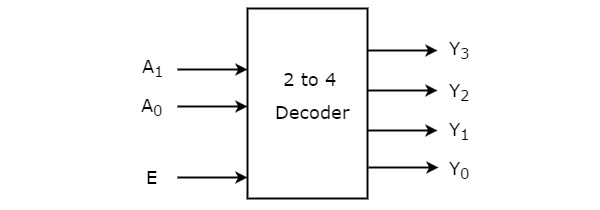Truth table

The Boolean functions for each output from the Truth table can written as follows:

The terms Y0, Y0, Y2, and Y3 are logically express as follows:

Y3=E.A1.A0

Y2=E.A1.A0′

Y1=E.A1′.A0

Y0=E.A1′.A0′

Circuit Diagram

The following is the logical circuit for the above expressions: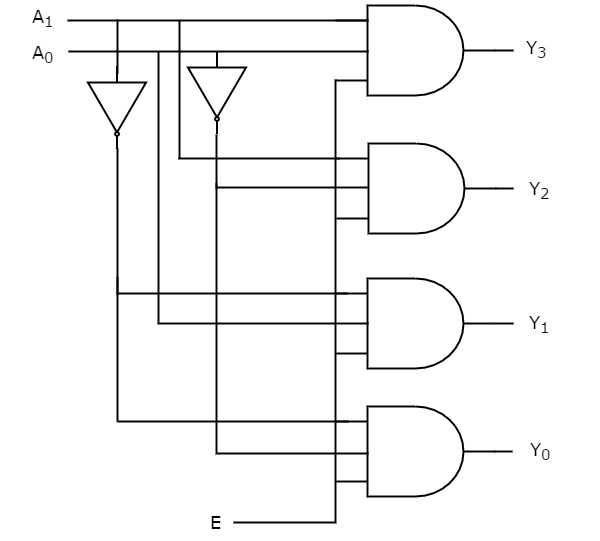## 3 to 8 line Decoder

Binary to Octal Decoder is another name for the 3 to 8 line decoder. A 3 to 8 line decoder has eight outputs, including Y0, Y1, Y2, Y3, Y4, Y5, Y6, and Y7, as well as three outputs, A0, A1, and A2. In this circuit, the enable input ‘E’ is utilize. When enable ‘E’ is set to 1, one of these four outputs will be 1, just like a two-to-four-line decoder. The block diagram and truth table for the 2 to 4 line decoder are presented below.

Block Diagram

Truth Table

A logical formulation for the terms D0, D1, D2, D3, D4, D5, D6, and D7 is as follows:

D0=A0′.A1′.A2′

D1=A0.A1′.A2′

D2=A0′.A1.A2′

D3=A0.A1.A2′

D4=A0′.A1′.A2

D5=A0.A1′.A2

D6=A0′.A1.A2

D7=A0.A1.A2

Circuit Diagram

The following is a logical circuit: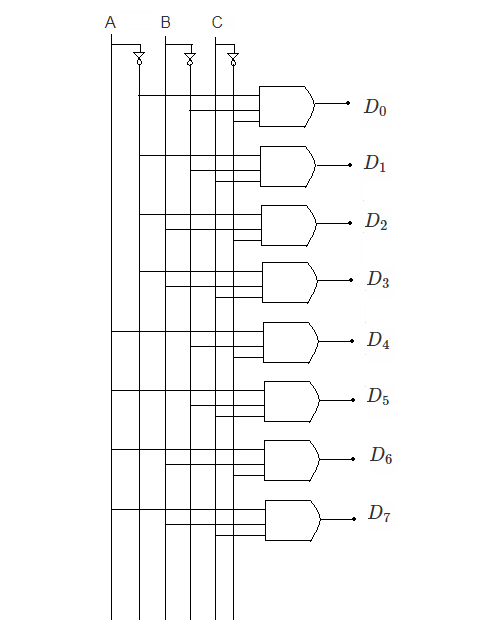## Application

• It use in the conversion of codes. This is analogue to digital conversion in the analogue decoder.
• It can also used to distribute data.
• In a high-performance memory system, this decode can utilized to lessen the burden of system decoding.
• As address decoders, the decoders are used in CPU memory location identification.
• It can also used to transform instructions into CPU control signals in electrical circuits.
• They’re mostly employed in data transport and logical circuits.
• They can also use to make simple digital logics such as half adders and full adders, as well as other digital designs.
• The microprocessor selects several I/O devices.

Interested in learning about similar topics? Here are a few hand-picked blogs for you!

The post What is Decoder? appeared first on Goseeko blog.

]]>
0
Bhumika <![CDATA[What is Encoder?￼]]> https://www.goseeko.com/blog/?p=7957 2022-05-25T12:06:44Z 2022-05-25T12:06:38Z The reverse operation of the Decoder is performed by an encoder, which is a combinational circuit.

The post What is Encoder?￼ appeared first on Goseeko blog.

]]>
The reverse operation of the Decoder is performed by an encoder, which is a combinational circuit. There are a total of 2n input lines and ‘n’ output lines. It will generate a binary code that is equivalent to the active High input. As a result, the encoder uses ‘n’ bits to encode 2n input lines. In encoders, representing the enable signal is optional.

## 4 to 2 line Encoder:

There are four inputs (Y0, Y1, Y2, and Y3) and two outputs (A0 and A1) in the 4 to 2 line encoder. In addition, To get the respective binary code on the output side, one input line at a time is set to true in a 4-input line. The 4 to 2 line encoder’s block diagram and truth table are shown below.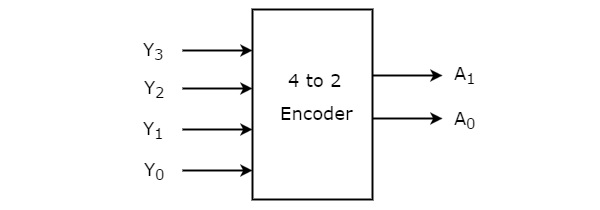Truth table

The terms A0 and A1 are logically expressed as follows:

A1=Y3+Y2

A0=Y3+Y1

Circuit Diagram

Two input OR gates can be used to implement the aforementioned two Boolean functions. Further, The 4 to 2 encoder circuit diagram is given in the graphic below.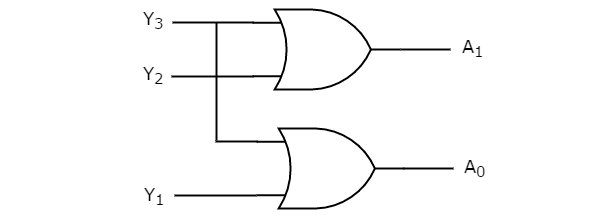## Octal to Binary Encoder

The octal to binary encoder has eight inputs, ranging from Y7 to Y0, and three outputs, A2, A1, and A0. The 8 to 3 encoder is the same as the octal to binary encoder. The following graphic depicts the block diagram of an octal to binary encoder.

Only one of these eight inputs can be ‘1’ at any given time in order to obtain the binary code. The octal to binary encoder’s truth table is presented below.

Truth Table

The following is the logical expression of the terms A0, A1, and A2:

A2=Y4+Y5+Y6+Y7

A1=Y2+Y3+Y6+Y7

A0=Y7+Y5+Y3+Y1

Circuit Diagram

The following is the logical circuit for the above expressions: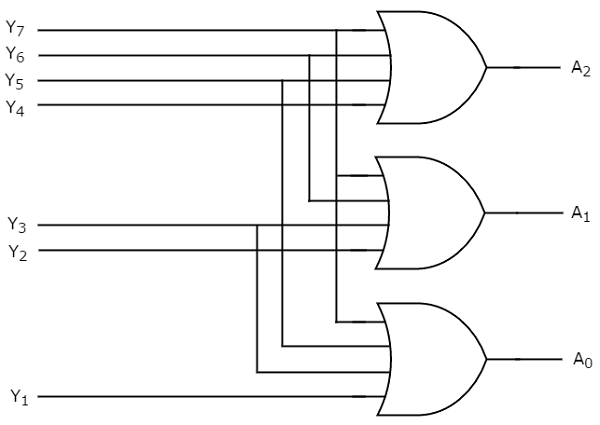## Uses of Encoder

In all digital systems, these systems are relatively simple to operate.

To convert a decimal number to a binary number, encoders are employed. The goal is to complete a binary operation like addition, subtraction, multiplication, and so on.

The disadvantages of a standard encoder are listed below.

• When all of the encoder’s outputs are 0, there is ambiguity. Because when only the least significant input is one or when all inputs are zero, it could be the code matching the inputs.
• When more than one input is set to high, the encoder generates an output that may or may not be the proper code. If both Y3 and Y6 are ‘1’, for example, the encoder outputs 111. This is neither the comparable code for Y3, when it is ‘1’, nor is it the equivalent code for Y6, when it is ‘1’.

Interested in learning about similar topics? Here are a few hand-picked blogs for you!

The post What is Encoder?￼ appeared first on Goseeko blog.

]]>
0
Sonali <![CDATA[What is Digital to Analog Converter?]]> https://www.goseeko.com/blog/?p=7944 2022-05-16T09:53:23Z 2022-05-16T09:53:23Z The Digital to Analog converter is an electronic device which converts digital signal to analog signal. Weighted Resistor and R-2R Ladder are its types.

The post What is Digital to Analog Converter? appeared first on Goseeko blog.

]]>
The Digital to Analog converter is an electronic device which converts digital signal to analog signal. The digital signals are in the form of 0 and 1. This converter produces only one output for multiple binary inputs. The number of binary inputs can be represented as 2^n where n is the number of binary inputs. The Weighted Resistor and R-2R Ladder are two types of Digital to Analog Converter.

## Weighted Resistor Digital to Analog Converter

This DAC uses binary weighted resistors to produce analog output equivalent to the binary input. The binary input is provided to the inverting adder circuit. Below figure represents the weighted resistor DAC. We can represent the binary number in the form of bits b0, b1, b2. The bit b0 is the least significant bit. The bit b1 is the most significant bit. The switches present will jump to ground when the input is equal to zero. When the input is 1 the switches will switch to position -VR which is the negative reference voltage.

The circuit consists of an Op-Amp which is an inverting op-amp as input is provided through the negative terminal. As we know from the virtual ground concept, the value of voltage at inverting and no-invertig end of the op-amp will be the same. Hence, the value of voltage at the input terminal node will be equal to 0V.

The value of current at each node can be determined by writing the nodal equations.

We have a 3-bit binary weighted resistor DAC. As there are only three input bits b0-b2. Then the number of possible outputs can be from 000 to 111 for a particular reference voltage VR.

Now for N-bit binary weighted resistor DAC the general form of output voltage can be

## R-2R Ladder DAC

This R-2R ladder DAC consists of R-2R ladder structure as shown below. Then the DAC produces analog output which is equal to the binary input. The ladder network then produces output with an inverting adder circuit in it. The figure below explains the circuit of R-2R ladder DAC.

Similar to Weighted Resistor DAC this circuit also has b0 as the least significant bit and b2 as the most significant bit. When the input bits are equal to 0 the digital switches jump to ground. When the input bits are 1 the switches jump to negative reference value -VR.

We can also easily find out the output voltage equation for this DAC for individual binary inputs. But it is very difficult to get the generalized output voltage equation in this case.

The reason why we prefer R-2R Ladder DAC over Weighted Resistor are

• The design of this type of DAC is easy as only R and 2R resistors are present.
• We can also include more R-2R combinations depending upon the required number of bits.

The post What is Digital to Analog Converter? appeared first on Goseeko blog.

]]>
0
krishna <![CDATA[What is a ring?]]> https://www.goseeko.com/blog/?p=7917 2022-05-16T09:53:23Z 2022-05-16T09:53:23Z A non-empty set R, equipped with two binary operations, called addition (+) and multiplication (.) is called a ring if the following…

The post What is a ring? appeared first on Goseeko blog.

]]>
A non-empty set R, equipped with two binary operations, called addition (+) and multiplication (.) is called a ring if the following postulates are satisfied.

(1) R is an additive Abelian group,

(2) R is an multiplicative semigroup

(3) The two distributive laws hold good, viz

a. (b + c) =a.b + a.c. (left distributive law)

(a + b). c = a.c + b.c, (right distributive law) for all a, b, c R

## Note:

1. In order to be a ring, there must be a non-empty set equipped with two binary operations, viz, + and . , satisfying the postulates mentioned above. Later on we will simply write ‘‘Let R be a ring’’. It should be borne in mind that there are two binary operations, viz, + and , on R.

2. It should also be borne in mind that the binary operations + and . may not be own usual addition and multiplication.

3. Since R is an additive Abelian group, the additive identity, denoted by 0, belongs to R. We call it the zero element of R. Here 0 is a symbol.

4. If a , bR, then – bR ( R is an additive group). a+ (– b) is, generally, denoted by a – b.

5. a.b is, generally, written as ab.

Commutative Ring: R is commutative if ab = ba, for all a, bR.

R with unity : R is said to be a ring with unity (or identity) if there exists an element e R such that

ae = a = ea, for all a R.

Note: A ring may or may not have a unity.

2. If R is a ring with unity, unity may be same as the zero element. For example, {0} is a commutative ring with unity. Here 0 is the additive as well as the multiplicative identity. It is known as the zero ring.

Interested in learning about similar topics? Here are a few hand-picked blogs for you!

The post What is a ring? appeared first on Goseeko blog.

]]>
0
krishna <![CDATA[What are the Increasing and decreasing functions?]]> https://www.goseeko.com/blog/?p=7907 2022-05-16T09:53:23Z 2022-05-16T09:53:23Z Introduction(Increasing and decreasing functions)- A function is said to be increasing function in an interval [a, b] if y increases as well…

The post What are the Increasing and decreasing functions? appeared first on Goseeko blog.

]]>
Introduction(Increasing and decreasing functions)- A function is said to be increasing function in an interval [a, b] if y increases as well as x increases from a to b.

Or in other words, A function is said to be an increasing function in [a, b] if its first derivative is greater than zero for all values of x in an interval [a, b].

And

A function is said to be decreasing function in an interval [a, b] if y decreases as well as x increases from a to b.

“If the first derivative is less than zero for all values of x in an interval [a, b] then the function y = f(x) is a decreasing function in [a, b].

## Maximum and minimum(Increasing and decreasing functions)

We call a function f(x) is maximum at x = a. If f(a) is greater than every other value of f(x) in the immediate neighbourhood of x = a. Then (i.e., f(x) ceases to increase but begins to increase at x = a.

Similarly, the minimum value of f(x) will be that value at x = b which is less than other values in the immediate neighbourhood of x = b.

## Maxima and minima of function of two variables

As we know that the value of a function at maximum point is the maximum value of a function.

Similarly, the value of a function at minimum point is the minimum value of a function.

The maxima and minima of a function is an extreme biggest and extreme smallest point of a function in a given range (interval) or entire region.

Pierre de Fermat was the first mathematician to discover general method for calculating maxima and minima of a function. The maxima and minima are complement of each other.

## Maxima and Minima of a function of one variables

If  f(x) is a single value function in a region R, then-

Maxima is a maximum point  if and only if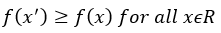Minima is a minimum point  if and only if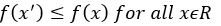The post What are the Increasing and decreasing functions? appeared first on Goseeko blog.

]]>
0
krishna <![CDATA[What is graph theory?]]> https://www.goseeko.com/blog/?p=7903 2022-05-16T09:53:23Z 2022-05-16T09:53:23Z Graph theory is intimately related to many branches of mathematics. It is widely applied in subjects like, Computer Technology, Communication Science, Electrical Engineering, Physics, Architecture, Operations Research, Economics, Sociology, Genetics, etc. In the earlier stages it was called slum Topology. It also has uses in social sciences, chemical sciences, information retrieval systems, linguistics even in economics also.

The post What is graph theory? appeared first on Goseeko blog.

]]>
Overview(graph theory)Graph theory is intimately related to many branches of mathematics. We widely use in subjects like, Computer Technology, Communication Science, Electrical Engineering, Physics, Architecture, Operations Research, Economics, Sociology, Genetics, etc..

It also has uses in social sciences, chemical sciences, information retrieval systems, linguistics even in economics also.

Graphs are discrete structures consisting of vertices and edges that connects these vertices.

There are several different types of graphs that differ with respect to the kind and number of edges that can a connect a pair of vertices.

## Basic terminology

Graph theory is a relatively new area of mathematics. Graph is the form of representing of descriptive data in the terms of verticals and edges. hence, we use Graph theory in various fields like computer science, information technology, genetics, telecommunication etc.

A graph is a collection of vertices and edges in which each edge is assigned to pair of points, we call them terminal.

Hence, we can say that a graph is a network of dots connect by lines.

Mathematically we can define a graph as-

A graph is a pair of set (V, E) where-

1. V is a non-empty set, we call its elements vertices.

2. E is collection of two-element subset of V, we call it edges.

Terminology: A graph G is an order pair (V, E) where V is a non-empty set and E is the set of edges in which each element of E is assign to a unique unorder pair of elements of V.

We denote an element of a set E as- e = (v, su) where u, v ∈ V.

hence, U and v are the end vertices of edge e.

Note- In any graph the number of vertices with odd degree must be even.

Loop: If both the end vertices of an edge are same then we call the edge as a loop.

Parallel edge: If two or more edges have same terminal vertices, then we call these edges as parallel edges.

Interested in learning about similar topics? Here are a few hand-picked blogs for you!

The post What is graph theory? appeared first on Goseeko blog.

]]>
0
krishna <![CDATA[What are matrices and their types?]]> https://www.goseeko.com/blog/?p=7901 2022-05-16T09:53:23Z 2022-05-16T09:53:23Z Matrices have a wide range of applications in various disciplines such as chemistry, Biology, Engineering, Statistics, economics, etc.

The post What are matrices and their types? appeared first on Goseeko blog.

]]>
Matrices have a wide range of applications in various disciplines such as chemistry, Biology, Engineering, Statistics, economics, etc. Matrices play an important role in computer science also. We widely use matrices to solve the system of linear equations, system of linear differential equations and nonlinear differential equations. First time the matrices were introduced by Cayley in 1860.

## Definition

A matrix is a rectangular arrangement of the numbers. We call these numbers inside the matrix as elements of the matrix.

HereThe expression of a matrix ‘A’ is-

The vertical elements are columns and the horizontal elements are rows of the matrix.

The order of matrix A is m by n or (m× n)

## Notation of a matrix

We denote a matrix ‘A’ is as-

Where, i = 1, 2, …….,m and j = 1,2,3,…….n

Here ‘i’ denotes row and ‘j’ denotes column.

## Types of matrices

1. Rectangular matrix-

A matrix in which the number of rows is not equal to the number of columns, then we say that the given matrix is a rectangular matrix.

Example:

The order of matrix A is 2×3, hence, it has two rows and three columns.

Matrix A is a rectangular matrix.

2. Square matrix-

A matrix with equal number of rows and columns, is a square matrix.

Example:

The order of matrix A is 3 ×3 , that means it has three rows and three columns.

Matrix A is a square matrix.

3. Row matrix-

A matrix with a single row and any number of columns is called row matrix.

Example:

4. Column matrix-

A matrix with a single column and any number of rows is called row matrix.

Example:

5. Null matrix (Zero matrix)-

A matrix in which each element is zero, then we call it a null matrix or zero matrix, therefore, we denote it by O.

Example:

6. Diagonal matrix-

A matrix is said to be diagonal matrix if all the elements except principal diagonal are zero

therefore, The diagonal matrix always follows-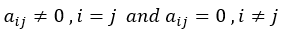So that, for Example: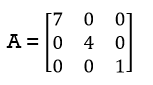7. Scalar matrix-

A diagonal matrix in which all the diagonal elements are equal to a scalar, hence, we call it a scalar matrix.

Example-

8. Identity matrix-

A diagonal matrix is said to be an identity matrix if its each element of diagonal is unity or 1.

Hence, we denote it by – ‘I’

9. Triangular matrix-

If every element above or below the leading diagonal of a square matrix is zero. therefore, we call it a triangular matrix.

Hence, there are two types of triangular matrices-

(a) Lower triangular matrix-

If all the elements below the leading diagonal of a square matrix are zero, therefore, we call it a lower triangular matrix.

Example:

(b) Upper triangular matrix-

If all the elements above the leading diagonal of a square matrix are zero, hence, we call it a lower triangular matrix.

Example –

The post What are matrices and their types? appeared first on Goseeko blog.

]]>
0